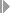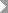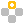Show TOC

###CFaR Single Value Analysis

You use this function to calculate Cash Flow at Risk online.

You can calculate the key figures for a portfolio hierarchy or for a specific portfolio hierarchy node as well as for a specific amount of financial objects (selected using different characteristics) independently of the portfolio hierarchies.

#### Activities

1. Call up the function in the Market Risk Analyzer application menu underInformation SystemCash Flow at RiskCFaR Single Value Analysis(transaction RMC0).

2. Select the mode in which you want to perform the program:

• CFaR Type

The evaluation parameters for calculating CFaR are taken from a CFaR type defined in Customizing.

If you select this mode, select first the Cash Flow at Risk type. Specify also the maturity band, the risk hierarchy (as well as any special risk hierarchy node), and the evaluation currency.

• CFaR Key Figure

The evaluation parameters for calculating CFaR are taken from a CFaR key figure defined for the results database (transaction AFWKF_RA).

Select a key figure with the key figure category RACFARC CFaR by Variance/Covariance or RACFARS CFaR from Simulation Method.

The settings Evaluation Type, Maturity Band, Risk Hierarchy, and Currency are taken from the key figure definition.

• CFaR Key Figure, Overwrite Settings

Similar to the option CFaR Key Figure. The settings Evaluation Type, Maturity Band, Risk Hierarchy, and Currency are taken from the key figure definition. However, you can change these settings on the selection screen.

3. On the Characteristics tab, you can use various characteristics from a broad range to select the financial objects to be analyzed.Example

• Company Code

• Transaction

• Product Category

• Product Type

• Position Currency

• ...

End of the example.
4. On the General Selections tab, you can restrict the selection of transactions to be analyzed by specifying the portfolio hierarchy and a portfolio hierarchy node.

5. Execute the program. The system calculates the desired key figures and displays them. The system does not store the key figures on the database.

CFaR and the cash flow are displayed aggregated across all selected financial objects for the individual periods of the maturity band as well as for the entire period.

• By selecting a period and then choosing the Toggle Transaction View <-> Aggregated pushbutton, you switch to the display screen showing the key figures at the level of the individual transactions.

• If you choose the Calculation Base pushbutton, the system displays the exchange rates used in the calculation.

• If you choose the Select Node in Risk Hierarchy pushbutton, you can display the key figures for a specific currency.

• For CFaR by variance/covariance, you can display the delta/gamma positions determined.

• For CFaR from Monte Carlo simulation, you can display the random walks (by choosing the Display Random Walks pushbutton) and the profits and losses determined (by choosing the P+L Distribution pushbutton).